# Learn Code By Gaming

Learn how to program by playing video games.

# OpenCV Object Detection in Games

### Grouping Rectangles into Click Points

May 17, 2020
Part of: OpenCV Object Detection in Games

Learn the trick to using OpenCV groupRectangles() for multiple object detection. This is part 3 in the OpenCV Python tutorial for gaming.

Grab the code on GitHub: https://github.com/learncodebygaming/opencv_tutorials
OpenCV Group Rectangles documentation: https://docs.opencv.org/4.2.0/d5/d54/group__objdetect.html#ga3dba897ade8aa8227edda66508e16ab9

In this part of the OpenCV tutorial series, I'm going to show you how to take overlapping results from `matchTemplate()` and turn them into single object detections. We'll do this by using OpenCV's groupRectangles(), and there is a trick I'll show you for using this function successfully. We'll finish up this section by converting our rectangle results into positions where our mouse could click to select the detected object.

So when we use thresholding with `matchTemplate()` and look at our results visually, we can quickly see that we've detected some number of objects. In the example below, it looks like we've detected 12 cabbages. But when we check our detected locations list, you'll see that we have far more than 12 results. This is because we've found many matching results that are very close to one another. You can also see this visually in the result image as some box lines look thicker. These are actually many different detection results all overlapping each other.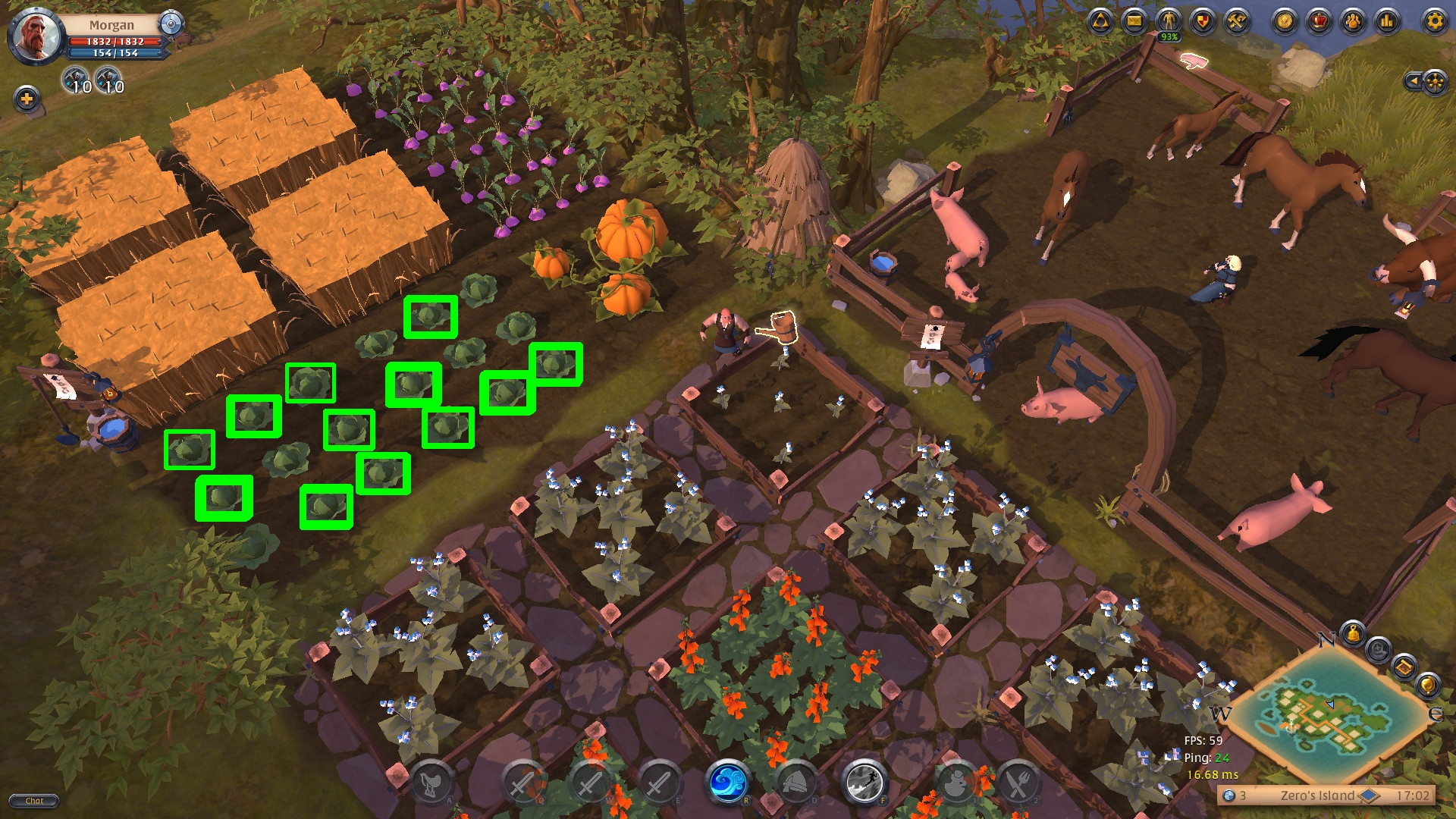We can solve this problem by using OpenCV's groupRectangles().

The `groupRectangles()` function expects a list of rectangles that are in the form of `[x, y, width, height]`. It will then return a new list of rectangles where the rectangles that are near to each other have been grouped together. So the first thing we must do to use this function is to convert our list of `(x, y)` location results into a list of rectangles.

``````needle_w = needle_img.shape
needle_h = needle_img.shape
rectangles = []
for loc in locations:
rect = [int(loc), int(loc), needle_w, needle_h]
rectangles.append(rect)
``````

Now that our rectangles list is constructed, we can call `groupRectangles()`:

``rectangles, weights = cv.groupRectangles(rectangles, groupThreshold=1, eps=0.5)``

The `groupThreshold` parameter will almost always be 1. If you set it to 0 it's not going to group any rectangles at all. And if you set it to something higher, it's going to require that more rectangles are overlapping each other before creating a grouped result for them.

The `eps` parameter controls how close together the rectangles need to be before they will be grouped together. Lower values require that rectangles be closer together to be merged, while higher values will group together rectangles that are farther away. This is a good value to play around with to make sure you're getting the results you expect.

Finally, `groupRectangles()` returns both the new rectangles list and weight information about the grouping process (which we will ignore).

If you run this code, you should find that your overlapping detection results have been discarded. But depending on the threshold that you set, you might also notice that some of your detections have been lost. Why is that?

This is the trick to using `groupRectangles()` that I mentioned earlier. If you have a lone match result that doesn't have any other nearby or overlapping matches to it, `groupRectangles()` will discard that result. The best way I've found to correct this issue is to simply add every rectangle to our rectangles list twice.

``````rectangles = []
for loc in locations:
rect = [int(loc), int(loc), needle_w, needle_h]
# Add every box to the list twice in order to retain single (non-overlapping) boxes
rectangles.append(rect)
rectangles.append(rect)
``````

This will give you back your lost results.

Now that we have nice detection results, where each object is detected just once, we can easily convert these rectangles into positions at the center of each rectangle. These represent points we could click on to select the detected object.

``````points = []
if len(rectangles):
# Loop over all the rectangles
for (x, y, w, h) in rectangles:
# Determine the center position
center_x = x + int(w/2)
center_y = y + int(h/2)
# Save the points
points.append((center_x, center_y))
# Draw the center point
cv.drawMarker(haystack_img, (center_x, center_y), color=(255, 0, 255), markerType=cv.MARKER_CROSS, markerSize=40, thickness=2)
``````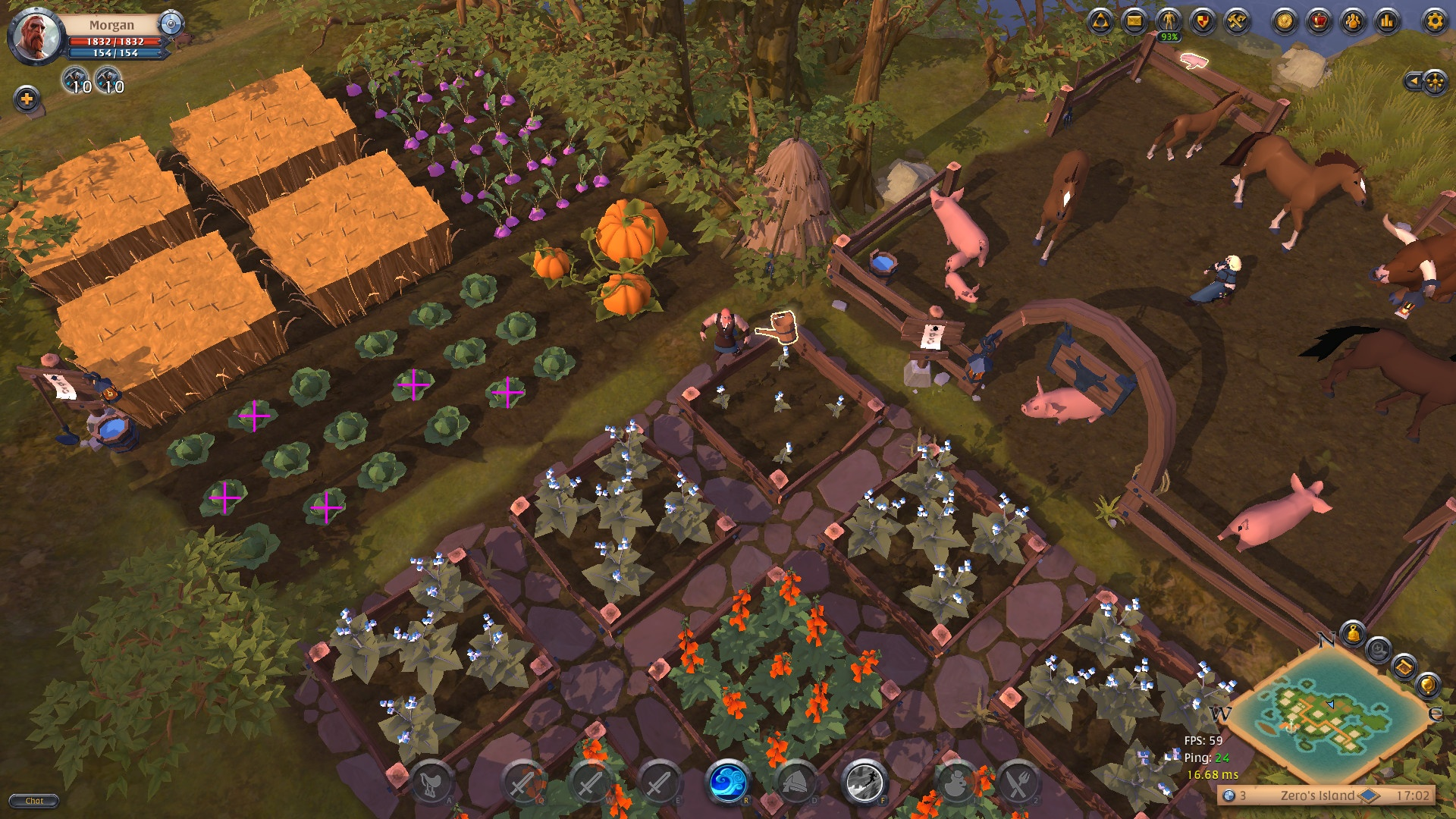To finish up this stage, let's take everything we've covered so far and wrap it up into a nice function. We'll have this function take a needle and haystack image as arguments, as well as the match threshold, and we'll have it return a list of object positions. We'll also make the debug output optional.

``````def findClickPositions(needle_img_path, haystack_img_path, threshold=0.5, debug_mode=None):

# Save the dimensions of the needle image
needle_w = needle_img.shape
needle_h = needle_img.shape

# There are 6 methods to choose from:
# TM_CCOEFF, TM_CCOEFF_NORMED, TM_CCORR, TM_CCORR_NORMED, TM_SQDIFF, TM_SQDIFF_NORMED
method = cv.TM_CCOEFF_NORMED
result = cv.matchTemplate(haystack_img, needle_img, method)

# Get the all the positions from the match result that exceed our threshold
locations = np.where(result >= threshold)
locations = list(zip(*locations[::-1]))
#print(locations)

# You'll notice a lot of overlapping rectangles get drawn. We can eliminate those redundant
# locations by using groupRectangles().
# First we need to create the list of [x, y, w, h] rectangles
rectangles = []
for loc in locations:
rect = [int(loc), int(loc), needle_w, needle_h]
# Add every box to the list twice in order to retain single (non-overlapping) boxes
rectangles.append(rect)
rectangles.append(rect)
# Apply group rectangles.
# The groupThreshold parameter should usually be 1. If you put it at 0 then no grouping is
# done. If you put it at 2 then an object needs at least 3 overlapping rectangles to appear
# in the result. I've set eps to 0.5, which is:
# "Relative difference between sides of the rectangles to merge them into a group."
rectangles, weights = cv.groupRectangles(rectangles, groupThreshold=1, eps=0.5)
#print(rectangles)

points = []
if len(rectangles):
#print('Found needle.')

line_color = (0, 255, 0)
line_type = cv.LINE_4
marker_color = (255, 0, 255)
marker_type = cv.MARKER_CROSS

# Loop over all the rectangles
for (x, y, w, h) in rectangles:

# Determine the center position
center_x = x + int(w/2)
center_y = y + int(h/2)
# Save the points
points.append((center_x, center_y))

if debug_mode == 'rectangles':
# Determine the box position
top_left = (x, y)
bottom_right = (x + w, y + h)
# Draw the box
cv.rectangle(haystack_img, top_left, bottom_right, color=line_color,
lineType=line_type, thickness=2)
elif debug_mode == 'points':
# Draw the center point
cv.drawMarker(haystack_img, (center_x, center_y),
color=marker_color, markerType=marker_type,
markerSize=40, thickness=2)

if debug_mode:
cv.imshow('Matches', haystack_img)
cv.waitKey()
#cv.imwrite('result.jpg', haystack_img)

return points
``````

Now we can easily use this to find objects in a variety of different images:

``````points = findClickPositions('albion_turnip.jpg', 'albion_farm.jpg', threshold=0.70, debug_mode='rectangles')
print(points)
``````

So far, we've been using OpenCV to detect objects in static images, and now we're ready to start applying the techniques we've learned in real time. But before we can do that, first we need a way to capture the screenshots for processing. In the next part of this series, I'm going to show you how you can capture 60 frames per second from any windowed application, even if it's on a second monitor or hidden behind other windows.###### Fast Window Capture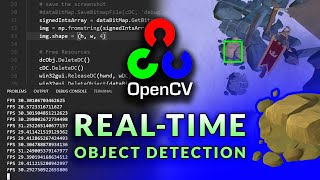###### Real-time Object Detection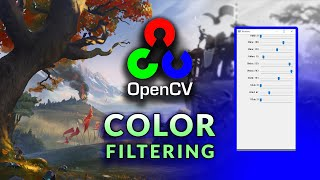###### HSV Color Range Thresholding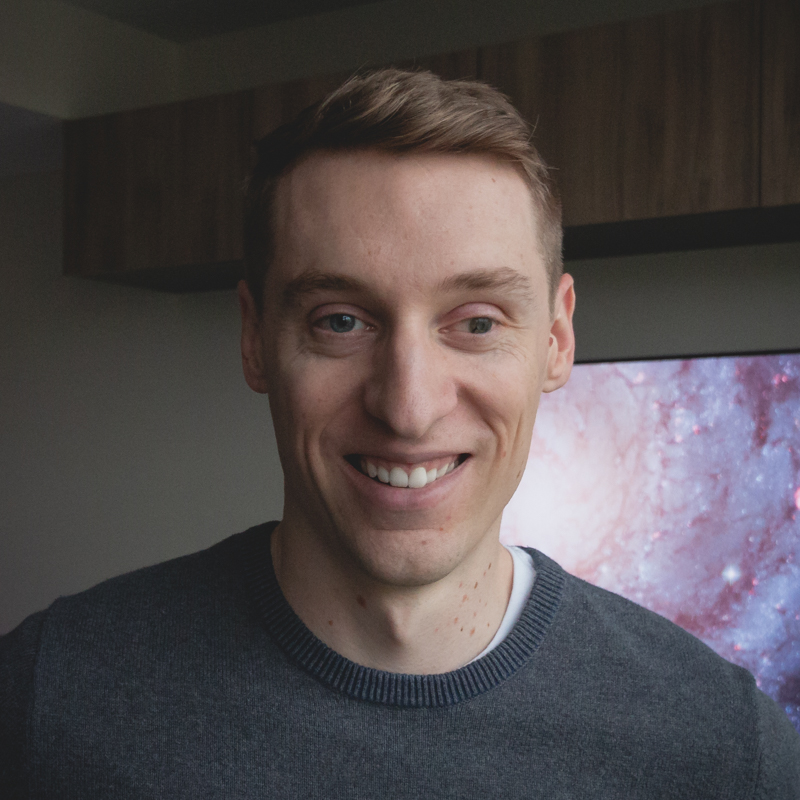My name is Ben and I help people learn how to code by gaming. I believe in the power of project-based learning to foster a deep understanding and joy in the craft of software development. On this site I share programming tutorials, coding-game reviews, and project ideas for you to explore.